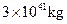Chapter 34, Problem 9PE

Chapter
Section
Textbook Problem

Assuming a circular orbit for the Sun about the center of the Milky Way galaxy, calculate its orbital Speed using the following information: The mass of the galaxy is equivalent to a single masstimes that at the Sun (orlocated 30,000 ly away.

To determine

The orbital speed.

Explanation

Given data:

Mass of sun is M=3×1041kg.

Gravitation constant is G=6.67×1011N.m2/kg2.

Radius of circular orbit is r=3000ly.

Formula used:

The formula for centrifigula force when sun moving around galxy is,

F1=mv2r   ......... (1)

The formula for gravitational force is,

F2=GMmr2   ......... (2)

According Newton second law,

mv2r=GMmr2

Rewrite the above equation for v.

v=( GMr)12

Still sussing out bartleby?

Check out a sample textbook solution.

See a sample solution

The Solution to Your Study Problems

Bartleby provides explanations to thousands of textbook problems written by our experts, many with advanced degrees!

Get Started